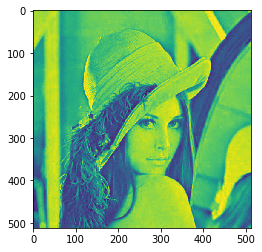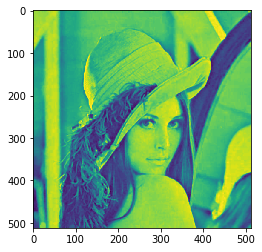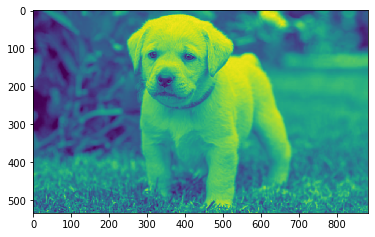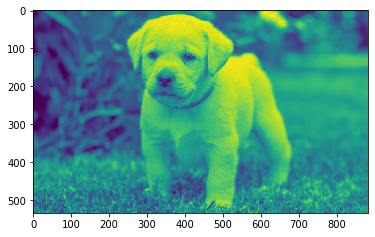GFG App
Open AppBrowser
Continue

# Mahotas – Median filter

In this article we will see how we can apply median filter to the image in mahotas. The median filter is a non-linear digital filtering technique, often used to remove noise from an image or signal. Such noise reduction is a typical pre-processing step to improve the results of later processing (for example, edge detection on an image).
In this tutorial we will use “lena” image, below is the command to load it.

`mahotas.demos.load('lena')`

Below is the lena imageIn order to do this we will use mahotas.median_filter method
Syntax : mahotas.median_filter(img)
Argument : It takes image object as argument
Return : It returns image object

Note : Input image should be filtered or should be loaded as grey
In order to filter the image we will take the image object which is numpy.ndarray and filter it with the help of indexing, below is the command to do this

`image = image[:, :, 0]`

Below is the implementation

## Python3

 `# importing required libraries` `import` `mahotas` `import` `mahotas.demos` `from` `pylab ``import` `gray, imshow, show` `import` `numpy as np` `import` `matplotlib.pyplot as plt` `  `  `# loading image` `img ``=` `mahotas.demos.load(``'lena'``)` `  `  `# filtering image` `img ``=` `img.``max``(``2``)`   `print``(``"Image"``)` `  `  `# showing image` `imshow(img)` `show()`   `# applying median filter` `new_img ``=` `mahotas.median_filter(img)` ` `    `# showing image` `print``(``"Median Filter"``)` `imshow(new_img)` `show()`

Output :

`Image``Median Filter`Another example

## Python3

 `# importing required libraries` `import` `mahotas` `import` `numpy as np` `from` `pylab ``import` `gray, imshow, show` `import` `os` `import` `matplotlib.pyplot as plt` ` `  `# loading image` `img ``=` `mahotas.imread(``'dog_image.png'``)`     `# filtering image` `img ``=` `img[:, :, ``0``]` `  `  `print``(``"Image"``)` `  `  `# showing image` `imshow(img)` `show()`   `# applying median filter` `new_img ``=` `mahotas.median_filter(img)` ` `    `# showing image` `print``(``"Median Filter"``)` `imshow(new_img)` `show()`

Output :

`Image``Median Filter`My Personal Notes arrow_drop_up